# GPoM : PreProcessing

## Pre-processing for global modelling

To apply the global modelling approach, a careful preprocessing may be necessary because time series derivatives are required during the modelling process. Derivatives will be computed automatically when using the gPoMo function. To avoid problems, a careful preprocessing should be applied beforehand to have more chance to obtain global models. This vignette aims to show simple examples of time series preprocessing for global modelling application. In particular, we show the usefulness to resample the data (in order to improve the quality of the derivatives) and some of the limitations that may be found, especially when considering derivatives of high degree.

In practice, other types of preprocessing may be necessary depending on the quality of the data set (time sampling and time series length length, level of noise, presence of gaps, etc.). The required preprocessing may also depend on other factors such as: the dimension of underlying dynamics, the number of observed time series, the degree of observability, etc.

## Single time series

When only one single time series is observed, a global model of canonical form may be expected:

$$dX_1/dt = X_2$$

$$dX_2/dt = X_3$$

$$...$$

$$dX_n/dt = F(X_1, X_2, ..., X_n)$$.

with $$X_1$$ the observed variable, $$X_2$$, $$X_3$$, etc. its successive derivatives. The GPoM package is based on polynomial formulations. For the present formulation, its aim is to obtain a polynomial approximation of the function $$F$$. An approximation of $$F$$ can be obtained using the gPoMo function (see vignette III_Modelling). In practice, the canonical form will require the computations of $$n$$ derivatives to have the following $$n + 1$$ time series: $$(X_1, X_2, ..., X_n, dX_n/dt)$$.

Chaotic systems are well adapted to illustrate the skills of the global modelling approach because they are not trivial study cases: they are nonlinear and their behavior is unpredictable at long term. The Rössler system introduced by Otto Rössler in 1976 is used here1. This system is defined as:

$$dx/dt = - y - z$$

$$dy/dt = x + a y$$

$$dz/dt = b + z (x - c)$$.

One of the interests of this system is to have different degrees of observability depending on the variable considered. This degree of observability results from the system nonlinearities. The question of observability is of first importance for global modelling since, depending on it, the model reconstruction may be easy, hard or completely impossible2. Time series of such equations can be easily generated with the GPoM package (see vignette I_Generate). A ready to use data set of the Rössler-1976 system is also provided in the package that can be directly loaded:

    # load data
data("Ross76")
# plot
tin <- Ross76[,1]
data <- Ross76[,2:4]
plot(tin, data[,1],
xlab = 't', ylab = 'x(t)', main = 'Original time series',
type='l', col = 'lightgray')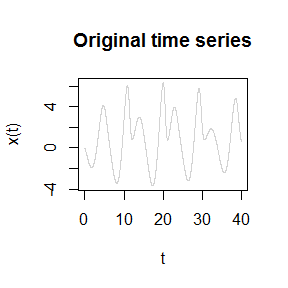The time series $$x(t)$$ here plotted shows an irregular behavior and will be used hereafter to illustrate the global modelling in a canonical form. To illustrate the problem of time sampling, the time series is undersampled as follows:

    plot(tin, data[,1],
xlab = 't', ylab = 'x(t)', main = 'Undersampled time series',
type='l', col = 'lightgray')
# subsampling:
Ross76us <- Ross76[seq(1,4000,by=50),]
# plot
tus <- Ross76us[,1]
datus <- Ross76us[,2:4]
lines(tus, datus[,1], type='p', col='red', cex = 0.6)
lines(tus, datus[,1], type='l', col='red')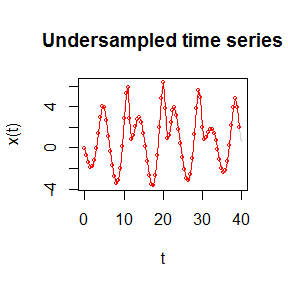The subsampling leads to loose some information. However, the general shape is obviously kept.

A spline can be used to resample the signal at the original sampling rate.

    plot(tin, data[,1],
xlab = 't', ylab = 'x(t)', main = 'Resampled time series',
type='l', col = 'lightgray')
# subsampling:
Ross76us <- Ross76[seq(1,4000,by=50),]
# plot
tus <- Ross76us[,1]
datus <- Ross76us[,2:4]
lines(tus, datus[,1], type='p', col='red', cex = 0.6)
lines(tus, datus[,1], type='l', col='red')
#
xout <- seq(min(tus), max(tus), by = 0.01)
rspl <- spline(tus, y = datus[,1], method = "fmm", xout = xout)
# plot resampled
lines(rspl$x, rspl$y, type='l', col='green')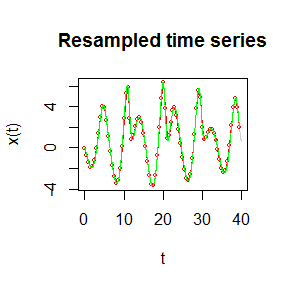In the present case, the spline resampling appears quite efficient to retrieve the original signal. Note that alternative methods may be preferred depending on the dynamical behavior considered, on the rate of subsampling, on the signal quality, etc.)

The drvSucc function of the GPoM package aims to estimate the derivatives. It is based on the algorithm introduced by Savitzky and Golay in 19643. This function is applied to the three time series presented upper: (1) the original Rössler time series, (2) its undersampling, and (3) its resampling to the original sampling using splines.

    # from original signal
drv1 <- drvSucc(tin, data[,1], nDeriv=2)
# from undersampled signal
drv2 <- drvSucc(tus, datus[,1], nDeriv=2)
# from resampled signal
drv3 <- drvSucc(rspl$x, rspl$y, nDeriv=2)

The obtained time series for the first and second derivatives are plotted hereafter. Obviously, much of the original signal (in black) is lost when computing the derivatives from the undersampled time series (in red). Contrarily, much of the original signal is retrieved when computed from the resampled signal (in green), which clearly illustate the usefulness to resample the signal with appropriate algorithms before the computation of the derivatives.

    # plot resulting output as a function of time
# output variable (smoothed) and its derivatives
#    plot(drv1$tout, drv1$seriesDeriv[,1], type='l', xlab = 'time', ylab = 'x(t)')
#    lines(drv2$tout, drv2$seriesDeriv[,1], type='p', cex = 0.6, col = 'red')
#    lines(drv3$tout, drv3$seriesDeriv[,1], type='l', col = 'green')
# first derivative
plot(drv1$tout, drv1$seriesDeriv[,2], type='l', xlab = 'time', ylab = 'dx(t)/dt')
lines(drv2$tout, drv2$seriesDeriv[,2], type='p', cex = 0.6, col = 'red')
lines(drv3$tout, drv3$seriesDeriv[,2], type='l', col = 'green')
# second derivative
plot(drv1$tout, drv1$seriesDeriv[,3], type='l', xlab = 'time', ylab = 'd²x(t)/dt²')
lines(drv2$tout, drv2$seriesDeriv[,3], type='p', cex = 0.6, col = 'red')
lines(drv3$tout, drv3$seriesDeriv[,3], type='l', col = 'green')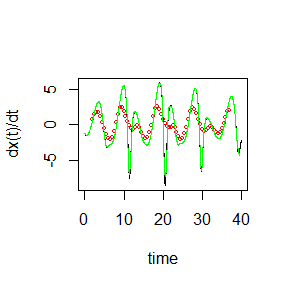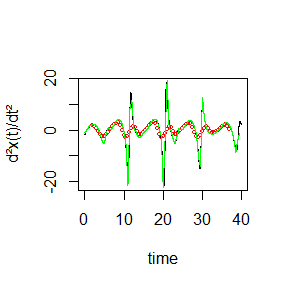Phase portraits can also be compared. Two projections are shown here after: $$(x, dx/dt)$$ and $$(x, d²x/dt²)$$. Obviously, quite much of the information of the original phase portraits (plotted in black) is lost when the phase portrait is reconstructed from the undersampled time series (in red). Contrarily, the projection $$(x, dx/dt)$$ of the phase portrait is retrieved almost perfectly when reconstructed from the resampled time series (in green). The second phase portrait $$(x, d²x/dt²)$$ shows that some drawbacks were introduced by the resampling, although this processing clearly improved the signal compared to the undersampled reconstruction.

    # output variable (smoothed) and its derivatives
plot(drv1$seriesDeriv[,1], drv1$seriesDeriv[,2], type='l', xlab = 'x(t)', ylab = 'dx(t)/dt')
lines(drv2$seriesDeriv[,1], drv2$seriesDeriv[,2], type='l', col = 'red')
lines(drv3$seriesDeriv[,1], drv3$seriesDeriv[,2], type='l', col = 'green')
# first derivative
#     plot(drv1$seriesDeriv[,1], drv1$seriesDeriv[,3], type='l', xlab = 'x(t)', ylab = 'd²x(t)/dt²')
#    lines(drv2$seriesDeriv[,1], drv2$seriesDeriv[,3], type='l', col = 'red')
#    lines(drv3$seriesDeriv[,1], drv3$seriesDeriv[,3], type='l', col = 'green')
# second derivative
plot(drv1$seriesDeriv[,2], drv1$seriesDeriv[,3], type='l', xlab = 'dx(t)/dt', ylab = 'd²x(t)/dt²')
lines(drv2$seriesDeriv[,2], drv2$seriesDeriv[,3], type='l', col = 'red')
lines(drv3$seriesDeriv[,2], drv3$seriesDeriv[,3], type='l', col = 'green')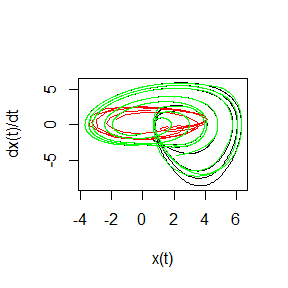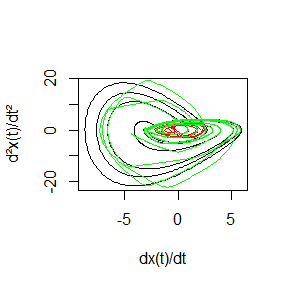This preprocessing illustrates (1) the usefulness to have a proper sampling of the observed process; (2) the usefulness to resample the observed time series at a better time sampling to improve the estimates of the derivatives; (3) some of the limitations reached for the derivatives of higher degree. Subsampling and resampling may have a direct impact when applying the global modelling techique and should thus be considered carefully when using the approach. Since the derivatives will be computed automatically when using the gPoMo function, it is important to keep in mind that their quality will directly depend on the characteristic of the time series provided as an input.

## Multiple time series

When several time series are available, global models of the following form can be expected:

$$dX_1/dt = f_1(X_1, X_2, ..., X_n)$$

$$dX_2/dt = f_2(X_1, X_2, ..., X_n)$$

$$...$$

$$dX_n/dt = f_n(X_1, X_2, ..., X_n)$$.

where the $$X_i$$, are the observed variables. The GPoM package can be used to obtain a polynomial approximation of the functions $$f_i$$ (see vignette III_Modelling). This formulation will require the computations of the first derivatives of each time series in order to have $$(X_1, X_2, ..., X_n, dX_1/dt, dX_2/dt, ... dX_n/dt)$$.

Here again the Rössler system (1976) is used to illustrate the skills of the global modelling approach.

    # load
data("Ross76")
# plot
tin <- Ross76[,1]
data <- Ross76[,2:4]
plot(tin, data[,1],
ylim = c(-6.5,12),
type='l', col='blue', xlab = 'time', ylab = 'x(t), y(t), z(t)')
lines(tin, data[,2], type='l',  col='orange')
lines(tin, data[,3], type='l',  col='brown')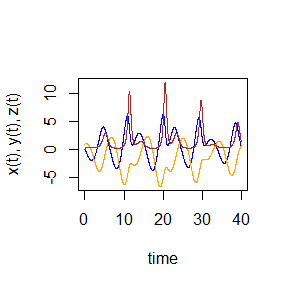The phase space of the original Rössler system can be reconstructed for the following set of time series $$x(t)$$, $$y(t)$$ and $$z(t)$$: The following reconstructions correspond to projections $$(x,y)$$ and $$(x,z)$$:

    plot(data[,1], data[,2], type='l', xlab = 'x(t)', ylab = 'y(t)')
#plot(data[,1], data[,3], type='l', xlab = 'y(t)', ylab = 'z(t)')
plot(data[,2], data[,3], type='l', xlab = 'x(t)', ylab = 'z(t)')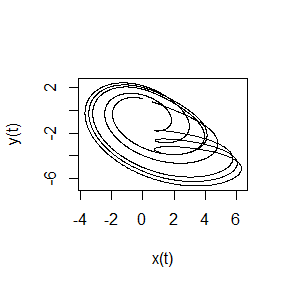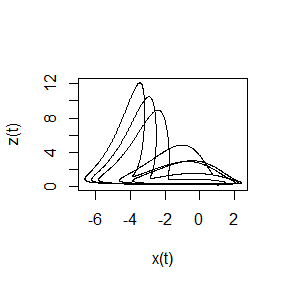For practical reasons, observations are often undersampled:

    # plot
tin <- Ross76[,1]
data <- Ross76[,2:4]
plot(tin, data[,1],
ylim = c(-6.5,12),
type='l', col='lightgray', xlab = 'time', ylab = 'x(t)')
lines(tin, data[,2], type='l',  col='lightgray', xlab = 'time', ylab = 'y(t)')
lines(tin, data[,3], type='l',  col='lightgray', xlab = 'time', ylab = 'z(t)')

# undersampled data
#
# subsampling:
Ross76us <- Ross76[seq(1,4000,by=75),]
# plot
tus <- Ross76us[,1]
datus <- Ross76us[,2:4]
lines(tus, datus[,1], type='p', cex = 0.5, col='blue')
lines(tus, datus[,2], type='p', cex = 0.5, col='orange')
lines(tus, datus[,3], type='p', cex = 0.5, col='brown')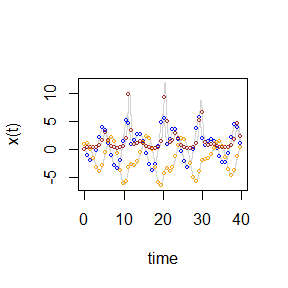Subsampling may lead to loose an important part of the original signal. This lost may vary from one observable to another (for example, several picks of the time series are missrepresented by the undersampled signal), and this effect will become stronger when considering derivatives of higher degrees (see the first Section of the present vignette). It can be useful in such case to resample the time series at a higher time resolution. Resampling can be performed with splines as exemplified upper for single time series. Alternative methods may be preferred depending on the complexity of the behavior under study, on the degree of subsampling, on the level of noise, etc. Here, this resampling appears efficient enough to obtain a good reconstruction of the phase space as illustrated by the following projections $$(x,y)$$ and $$(x,z)$$.

    xout <- seq(min(tus), max(tus), by = 0.01)
rsplx <- spline(tus, y = datus[,1], method = "fmm", xout = xout)
rsply <- spline(tus, y = datus[,2], method = "fmm", xout = xout)
rsplz <- spline(tus, y = datus[,3], method = "fmm", xout = xout)
# plot resampled
plot(data[,1], data[,2], type='l', xlab = 'x(t)', ylab = 'y(t)')
lines(rsplx$y, rsply$y, type='l', col='green')
#    plot(data[,1], data[,3], type='l', xlab = 'y(t)', ylab = 'z(t)')
#    lines(rsplx$y, rsplz$y, type='l', col='green')
plot(data[,2], data[,3], type='l', xlab = 'x(t)', ylab = 'z(t)')
lines(rsply$y, rsplz$y, type='l', col='green')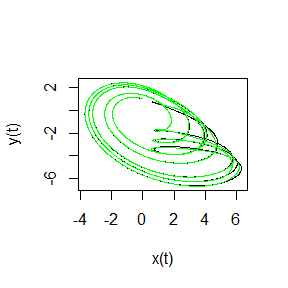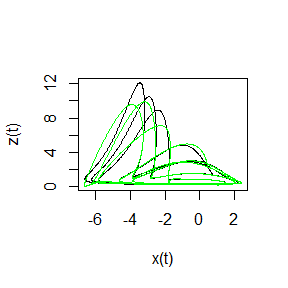Discrepancies related to the derivatives can be easily observed considering the derivative phase portraits. The skills of resampling is obviously good for variable $$y$$:

    # derivatives
# from original signal
drv1x <- drvSucc(tin, data[,1], nDeriv=2)
drv1y <- drvSucc(tin, data[,2], nDeriv=2)
drv1z <- drvSucc(tin, data[,3], nDeriv=2)
# from resampled signal
drv3x <- drvSucc(rsplx$x, rsplx$y, nDeriv=2)
drv3y <- drvSucc(rsply$x, rsply$y, nDeriv=2)
drv3z <- drvSucc(rsplz$x, rsplz$y, nDeriv=2)
# phase portraits plot for y:
# (y, dy/dt) projection of the original signal (in black) and resampled signal (in green)
plot(drv1y$seriesDeriv[,1], drv1y$seriesDeriv[,2], type='l', xlab = 'y', ylab = 'dy/dt')
lines(drv3y$seriesDeriv[,1], drv3y$seriesDeriv[,2], type='l', col='green')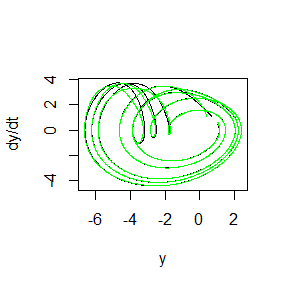Resampling appears less efficient for variables $$x$$ and $$z$$:

    # phase portraits plot for x and z:
# (x, dx/dt) projection of the original signal (in black) and resampled signal (in green)
plot(drv1x$seriesDeriv[,1], drv1x$seriesDeriv[,2], type='l', xlab = 'x', ylab = 'dx/dt')
lines(drv3x$seriesDeriv[,1], drv3x$seriesDeriv[,2], type='l', col='green')
# phase portraits plots
# (z, dz/dt) projection of the original signal (in black) and resampled signal (in green)
plot(drv1z$seriesDeriv[,1], drv1z$seriesDeriv[,2], type='l', xlab = 'z', ylab = 'dz/dt')
lines(drv3z$seriesDeriv[,1], drv3z$seriesDeriv[,2], type='l', col='green')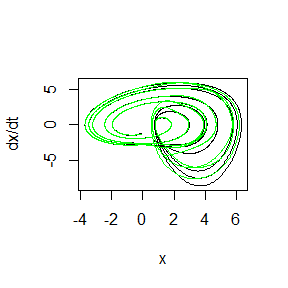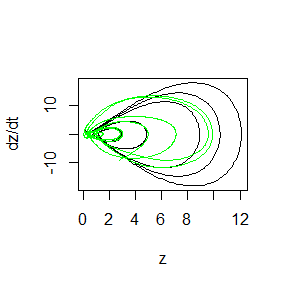Although resampling with splines may not permit to reconstruct the original signal perfectly, from a practical point of view, it appears necessary in many cases, and it is in practice a rather efficient way to deal with subsampling. This type of resampling could be efficiently used for applying the global modelling technique both from single time series4, and multiple time series5 6.

## Next step

Once the time series have been carefuly prepared, the global modelling technique can be tried. This is presented in the next vignette III_Modelling.

1. O. Rössler, 1976. An Equation for Continuous Chaos, Physics Letters, 57A(5), p. 397-398.

2. C. Letellier, L. A. Aguirre, & J. Maquet, 2005. Relation between observability and differential embeddings for nonlinear dynamics, Phys. Rev. E 71(6), 066213.

3. A. Savitzky & M. J. Golay, 1964. Smoothing and differentiation of data by simplified least squares procedures, Anal. Chem. 36(8), 1627–1639.

4. S. Mangiarotti, L. Drapeau, & C. Letellier, 2014. Two chaotic global models for cereal crops cycles observed from satellite in Northern Morocco, Chaos, 24, 023130.

5. S. Mangiarotti, 2015. Low dimensional chaotic models for the plague epidemic in Bombay (1896–1911), Chaos, Solitons, Fractals 81(A), 184–196.

6. S. Mangiarotti, M. Peyre & M. Huc, 2016. A chaotic model for the epidemic of Ebola virus disease in West Africa (2013–2016). Chaos, 26, 113112.